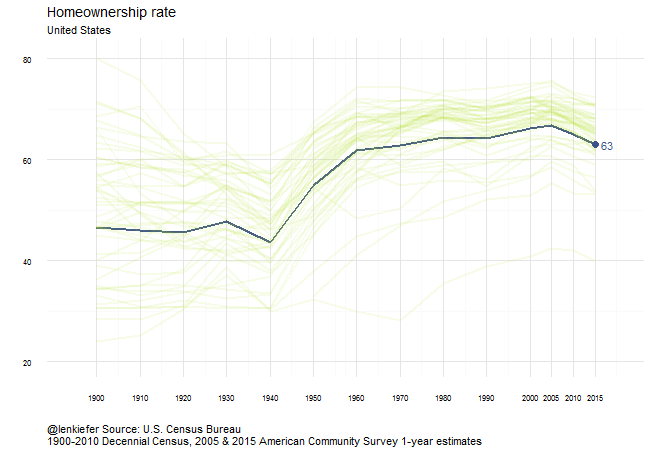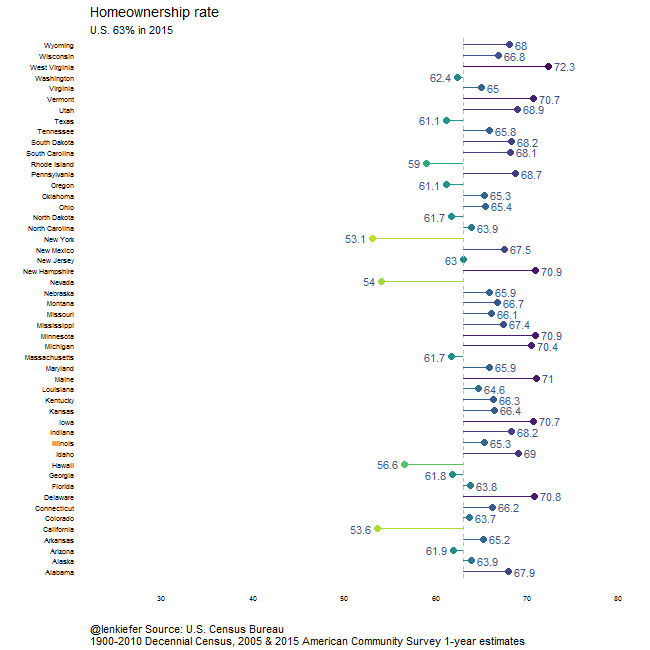# Visualizing the history of U.S. homeownership rates

IN THIS POST I WANT TO LOOK AT THE HISTORY OF HOMEOWNERSHIP in the United States. I’m going to go relatively far back in time, using Census data to compare the evolution of the homeownership rate by state from 1900 through 2015. Along the way we’ll make several different visualizations.

## Data

For data we’re going to rely on the U.S. Census Bureau. This page has a tabulation of historical homeownership rates from 1900 through 2000. Then we’ll supplement these estimates with estimates from the 2010 Decennial Census and the 2005 and 2015 American Community Survey, which can be downloaded from Census here.

I’ve put all the data into a single long text file with columns for state, year, and the value of the homeownership rate. You can download these data in a text file here.

# Let’s make some graphs

First let’s set up some libraries and take a peek at our data.

{% highlight r library(viridis) library(data.table) library(ggplot2) library(scales) library(tweenr) library(animation) library(dplyr) library(viridis) library(“htmlTable”) ho.dat<-fread(“data/horate.txt”) #load data

# view data:

htmlTable(head(ho.dat), col.rgroup = c(“none”, “#F7F7F7”),caption=“Homeownership data:“, tfoot=“horate.txt”) {% endhighlight

 state year value Homeownership data: 1 United States 2010 65.1 2 United States 2000 66.2 3 United States 1990 64.2 4 United States 1980 64.4 5 United States 1970 62.9 6 United States 1960 61.9 horate.txt

Now we’ll plot the U.S. homeownership rate:

{% highlight r ho.dat$st<-factor(ho.dat$state,levels=unique(ho.dat$state)) #make a factor out of the state variable for use later ggplot(data=ho.dat[state==“United States”,],aes(x=year,y=value))+ geom_line(color=viridis(5),size=1.05)+ theme_minimal()+ geom_text(data=ho.dat[state==“United States” & year==2015,],hjust=0,aes(label=paste(” “,round(value,1))),color=viridis(5))+ geom_point(data=ho.dat[state==“United States” & year==2015,],color=viridis(5),size=3)+ scale_x_continuous(breaks=unique(ho.dat$year))+ scale_y_continuous(breaks=seq(40,70,5))+ coord_cartesian(xlim=c(1895,2020))+ labs(x=“”,y=“”,title=“Homeownership rate (%)”, subtitle=“United States”, caption=“@lenkiefer Source: U.S. Census Bureau\n1900-2010 Decennial Census, 2005 & 2015 American Community Survey 1-year estimates”)+ theme(plot.caption=element_text(hjust=0))+ theme(axis.text=element_text(size=7)) {% endhighlightThis chart shows the decline in the homeownership rate since 2005 for the United States. At 63% the level of homeownership is the lowest in several decades. The Housing Vacancy Survey has updated estimates through the second quarter of 2016 and the rate of 62.9 percent in 2016Q2 is the lowest since the start of the survey in 1965.

However, the longer history that we can get from the Decennial Census shows us that the homeownership rate has been much lower before the 1960s.

## Homeownership rates by state

We can also look at the rate of homeownership by state. Let’s try several different ways to examine it.

### Panel plot

Let’s compare the homeownership rate by state using small multiples. So we have 50 states, I’m going to drop the District of Columbia. For each state we’ll plot a line chart using the historical rates of homeownership for each state.

{% highlight r ggplot(data=ho.dat[! (state %in% c(“United States”,“District of Columbia”)),],aes(x=year,y=value))+ geom_line(color=viridis(5),size=.95)+ theme_minimal()+ geom_text(data=ho.dat[! (state %in% c(“United States”,“District of Columbia”)) & year==2015,],hjust=0,aes(label=paste(” “,round(value,0))),color=viridis(5),size=2)+ geom_point(data=ho.dat[! (state %in% c(“United States”,“District of Columbia”)) & year==2015,],color=viridis(5),size=1)+ scale_x_continuous(breaks=c(1900,2015))+ scale_y_continuous(breaks=seq(20,80,20))+ coord_cartesian(xlim=c(1895,2025))+facet_wrap(~state,ncol=5)+ labs(x=“”,y=“”,title=“Homeownership rate (%)”, subtitle=“by state”, caption=“@lenkiefer Source: U.S. Census Bureau\n1900-2010 Decennial Census, 2005 & 2015 American Community Survey 1-year estimates”)+ theme(plot.caption=element_text(hjust=0))+ theme(axis.text=element_text(size=7)) {% endhighlightThe chart shows that most states follow a pattern similar to the U.S., the the rate of increase and post-2005 decline varies by state.

### Dot plot

An alternative to the line plot is a dot plot, which compares the level of homeownership across states at a particular point in time. For example, the graph below compares the homeownership rate in 2015 across states:

{% highlight r ggplot(data=ho.dat[! (state %in% c(“United States”,“District of Columbia”)) & year==2015,],aes(y=reorder(state,-value),x=value,color=value))+ scale_color_viridis(direction=-1,end=0.9)+ geom_point(size=3)+geom_vline(xintercept=63,color=“gray”,linetype=2)+ theme_minimal()+ annotate(geom=“text”,x=63,y=50,label=” U.S.:63%“,hjust=0)+ theme(legend.position=“none”)+ #suppress color legend theme(panel.grid.major = element_blank(), panel.grid.minor = element_blank())+ #remove gridlines geom_segment(aes(xend=63,x=value,yend=reorder(state,-value)))+ #add segment from U.S. average

geom_text(aes(label=paste(” “,round(value,1),” “),hjust=ifelse(value>63,0,1)),color=viridis(5),size=4)+ scale_x_continuous(limits=c(50,75),breaks=seq(50,75,5))+ labs(x=“”,y=“”,title=“Homeownership rate (%) in 2015”, subtitle=“”, caption=“@lenkiefer Source: U.S. Census Bureau\n1900-2010 Decennial Census, 2005 & 2015 American Community Survey 1-year estimates”)+ theme(plot.caption=element_text(hjust=0))+ theme(axis.text=element_text(size=7)) {% endhighlightThe plot shows that while the U.S. homeownership rate averaged 63 percent in 2015, there’s substantial variation across states. California and New York, with large populations of renters and high housing costs have the homeownership rates below 54%. Eight states (DE, IA, ME, MI, MN, NH, VT, and WV) had a homeownership rate over 70 percent in 2015.

## Animated plots

We can use tweenr to create animated versions of these plots to see how homeownership rates have varied over time and across states.

### Spaghetti plotThis plot compares each state’s time series. The backgroud “spaghetti” plot is an individual line for each state. We then cycle through each state and highlight them in turn.

### Lollipops

We can also have dancing lollipops, by animating the dot plot to cycle through each year:This chart shows that the dispersion of homeownership rates around the U.S. average has varied quite a lot over history.

## Animation Code

Code for the animations can be found below. I’m using the R package tweenr to make the animations.

See my earlier post about tweenr for an introduction to tweenr, and more examples here and here.

{% highlight r

## make the time series plot

myf<-function(s){as.data.frame(ho.dat[state==s, list(year,st,value)])}

# use lapply to generate the list of data sets:

my.list<-lapply(c(st.list,st.list),myf)

tf <- tween_states(my.list, tweenlength= 2, statelength=3, ease=rep(‘cubic-in-out’,24), nframes=300) tf<-data.table(tf) oopt = ani.options(interval = 0.1) saveGIF({for (i in 1:max(tf$.frame)) { my.state<-unique(tf[.frame==i]$st)

g<- ggplot(data=tf[.frame==i,],aes(x=year,y=value))+ geom_line(color=viridis(5),size=1.05)+ # faint lines for states not featured: geom_line(data=ho.dat,color=viridis(10),size=1.05,alpha=0.1,aes(x=year,y=value,group=st))+ geom_text(data=tf[.frame==i & year==2015,],hjust=0,aes(label=paste(” “,round(value,1))),color=viridis(5))+ geom_point(data=tf[.frame==i & year==2015,],color=viridis(5),size=3)+ theme_minimal()+ scale_x_continuous(breaks=unique(ho.dat$year))+ coord_cartesian(ylim=c(20,81),xlim=c(1895,2020))+ #set limites labs(x=“”,y=“”,title=“Homeownership rate”, subtitle=my.state, caption=“@lenkiefer Source: U.S. Census Bureau\n1900-2010 Decennial Census, 2005 & 2015 American Community Survey 1-year estimates”)+ theme(plot.caption=element_text(hjust=0))+ theme(axis.text=element_text(size=8)) print(g) ani.pause() } },movie.name=“ho rate by state viz.gif”,ani.width = 650, ani.height = 450) ho.dat$yf<-factor(ho.dat$year) #create year factor for plots ### make the panel plot myf2<-function(y){as.data.frame(ho.dat[year==y, list(yf,st,value)])} # use lapply to generate the list of data sets: my.list2<-lapply(c(2015,seq(1900,2000,10),2005,2010,2015),myf2) tf <- tween_states(my.list2, tweenlength= 2, statelength=3, ease=rep(‘cubic-in-out’,24), nframes=200) tf<-data.table(tf) oopt = ani.options(interval = 0.1) saveGIF({for (i in 1:max(tf$.frame)) { us.v<-unique(tf[.frame==i & st==“United States”]$value) g<- ggplot(data=tf[.frame==i & !(st %in% c(“United States”,“District of Columbia”)),],aes(y=st,x=value,color=value))+ scale_color_viridis(direction=-1,end=0.9)+ geom_point(size=3)+ geom_vline(xintercept=us.v,color=“gray”,linetype=2)+ theme_minimal()+ #annotate(geom=“text”,x=us.v,y=0,label=paste(” U.S.:“,us.v,”%“),hjust=0)+ theme(legend.position=“none”)+ #suppress color legend theme(panel.grid.major = element_blank(), panel.grid.minor = element_blank())+ #remove gridlines geom_segment(aes(xend=us.v,x=value,yend=st))+ #add segment from U.S. average geom_text(aes(label=paste(” “,round(value,1),” “),hjust=ifelse(value>us.v,0,1)),color=viridis(5),size=4)+ scale_x_continuous(limits=c(25,80),breaks=seq(30,80,10))+ labs(x=“”,y=“”,title=“Homeownership rate”, subtitle=paste0(“U.S. “,round(us.v,0),”% in “,unique(tf[.frame==i,]$yf)), caption=“@lenkiefer Source: U.S. Census Bureau\n1900-2010 Decennial Census, 2005 & 2015 American Community Survey 1-year estimates”)+ theme(plot.caption=element_text(hjust=0))+ theme(axis.text=element_text(size=7))

print(g) ani.pause() print(i) } },movie.name=“ho rate by state viz 2.gif”,ani.width = 650, ani.height = 650) {% endhighlight